# joxin-asemic

## Asemic Alphabet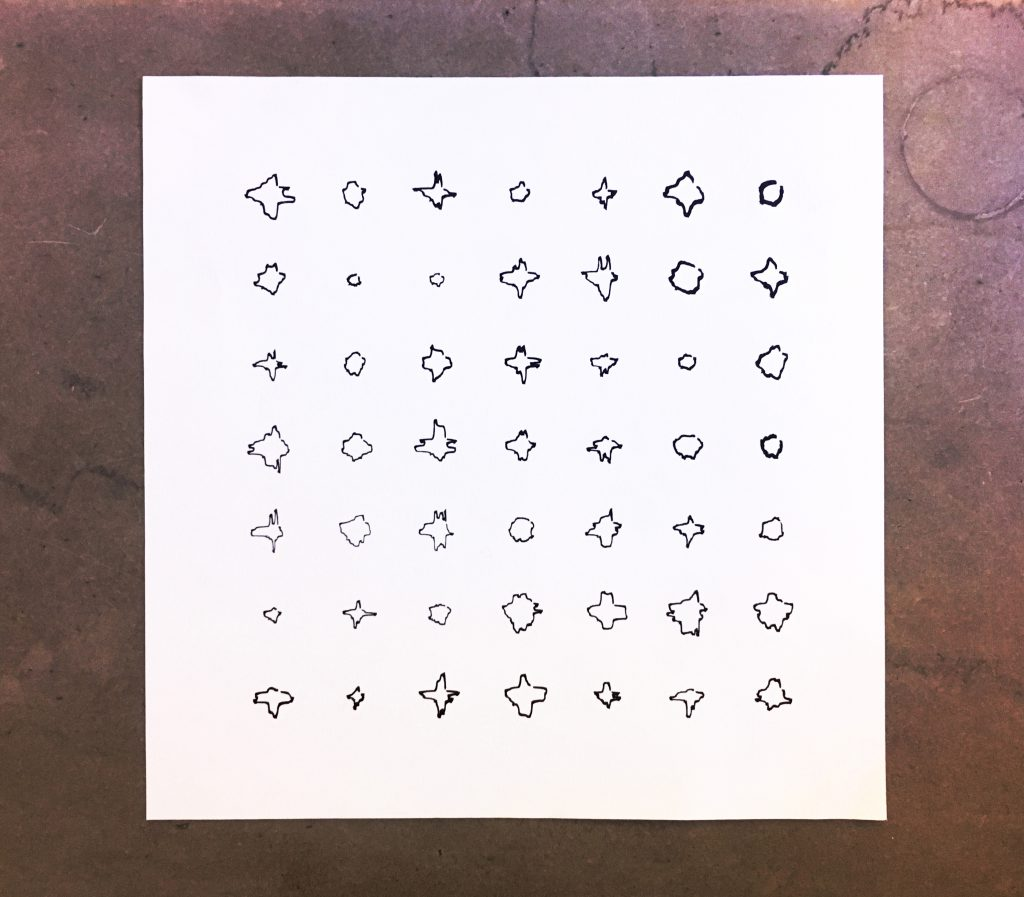A visual language system is a synthesis of rules and room for improvisation. For my own asemic language, I wanted to design the form of each character based on consistent rules in geometry and then generate possibilities using randomness.

To design the rules for generating characters, I began with basic shapes. I played around with circles for a while but wasn’t happy with how it looked. So I tried squares.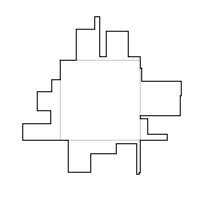In each character, “spikes” with straight edges come out of a square. The spikes vary in number and in size.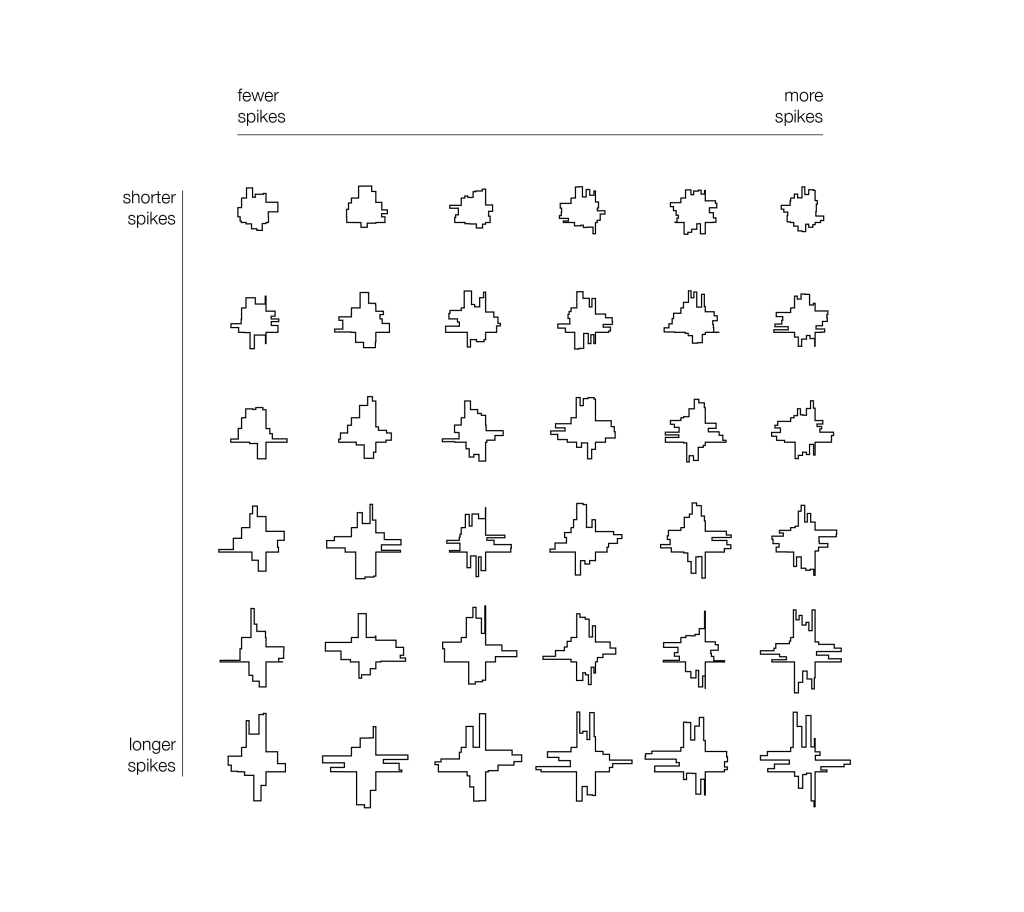The spikes differentiate the characters from each other. For the creatures from the other universe who would use this language, I imagine, the size and number of the spikes signify special meanings, sentiments, and conventions.

A paragraph of this language may look like this: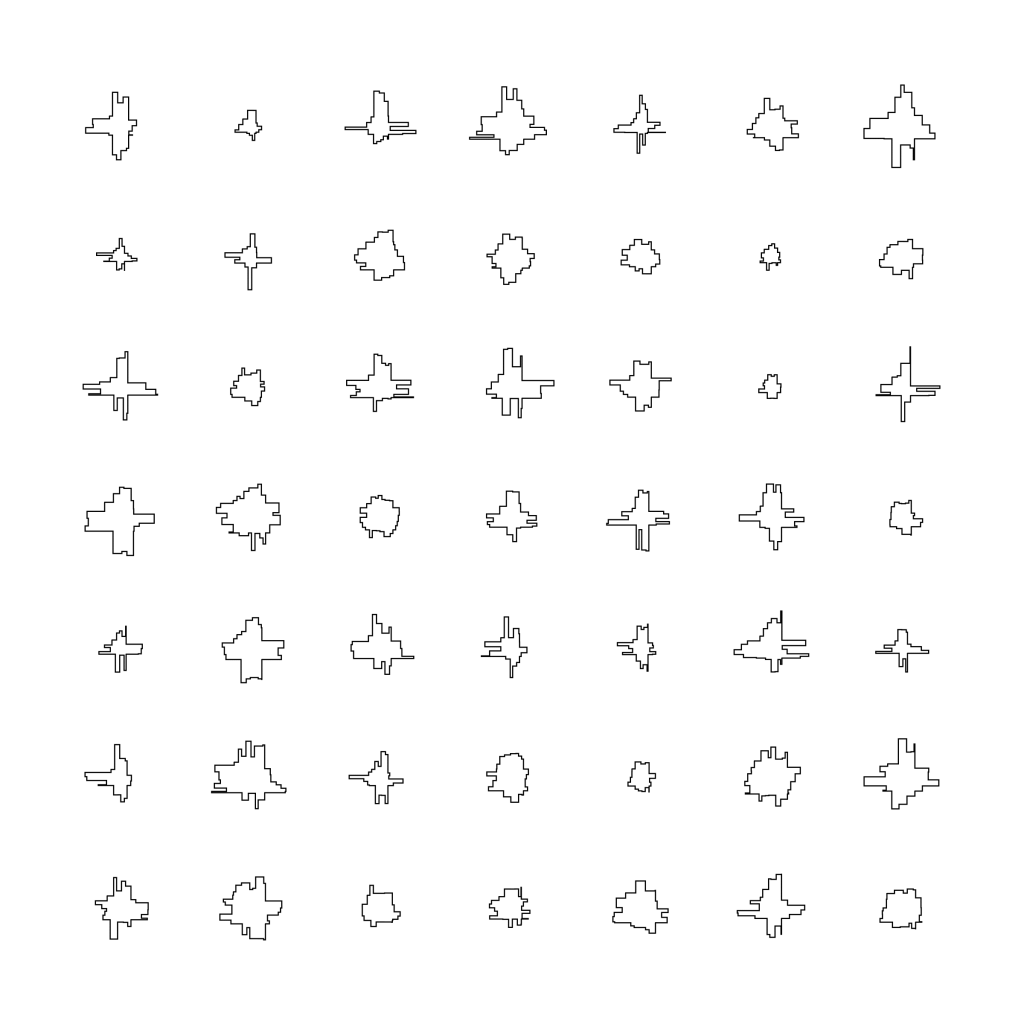When I look at this design in Processing, the characters have a very geometric, mechanical feel, because all the angles are 90 degrees. It feels more like an encrypted series of code, rather than language. Language is intriguing and infinite because it is expressive and unpredictable. It has a level of ~flare~.

To add an element of surprise, I decided to use a brush pen to plot it on Axidraw.
The brush pen gives very different effects depending on the speed of the plotter, and by how much it is being pressed down on the paper. The bleeding and the changing stroke weight smooth out the sharp corners in the original Processing design. When we plot huge characters, the details become more defined, but the smoothing effects also decrease.

I played around with paper of different colors as well.Eventually, I decided to plot fast, draw small characters, and use white paper, because I thought it gave the best result for smoothening out sharp edges, highlighting general form, and creating a calligraphic feel. I am happy that the brush pen helped deviate from the very geometric, mechanical look, and makes the writing system look more life-like.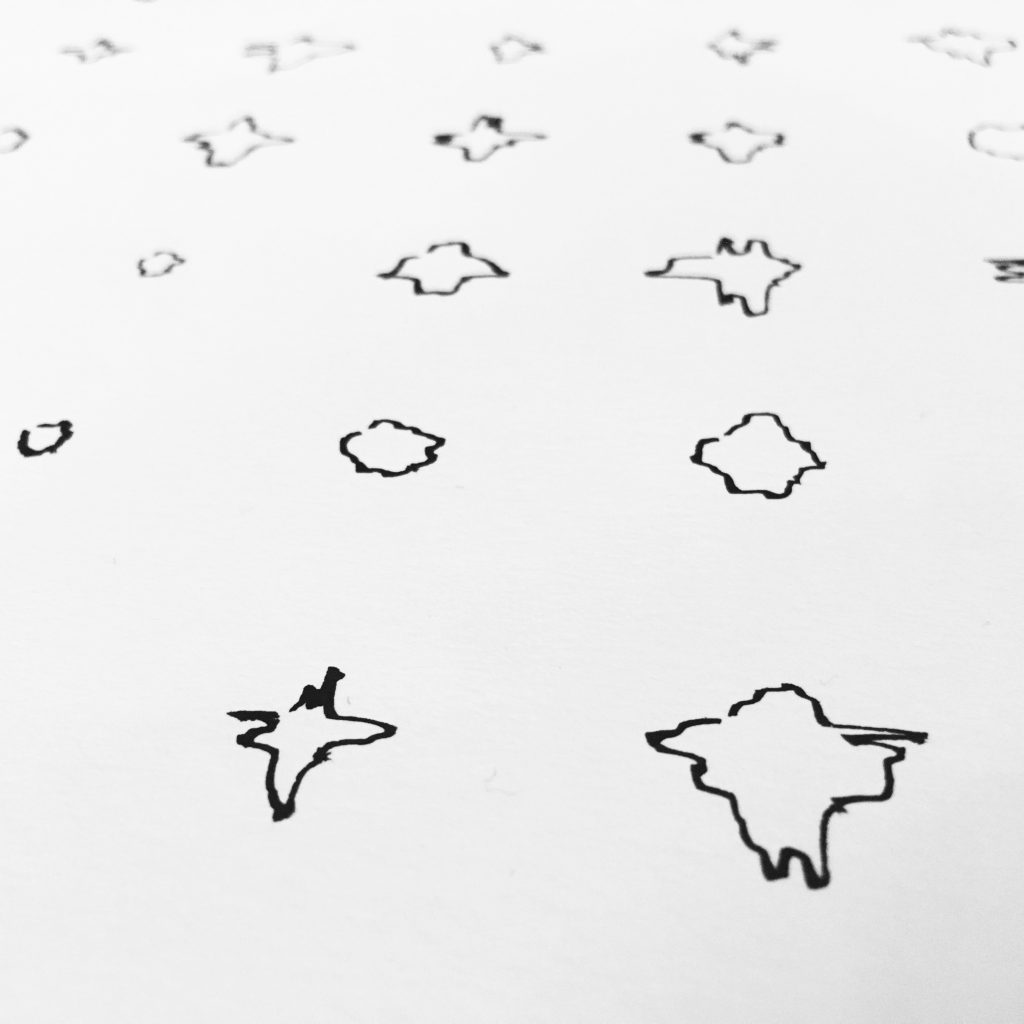I enjoyed the process of this project very much, because I got to practice paying close attention to form, which is not something I am very good at. I was surprised by how many variables can stem from a simple premise—size of the square, spacing, organization of the page, writing instrument, paper—every choice generates a new set of possibilities, every one of which needs consideration. I wanted to make something that look good. No matter whether the result actually looks good or not, I am glad I got to think a TON about what “looking good” really means in the process.

I am imagining…the creatures who use this system might look like this. They have spikes coming out of their face hole, which reorganize and write the squares and spikes.~Special thanks to Claire Hentschker for helping me with the plotter, and cambu for giving me input on documentation

My code:

```1 2 3 4 5 6 7 8 9 10 11 12 13 14 15 16 17 18 19 20 21 22 23 24 25 26 27 28 29 30 31 32 33 34 35 36 37 38 39 40 41 42 43 44 45 46 47 48 49 50 51 52 53 54 55 56 57 58 59 60 61 62 63 64 65 66 67 68 69 70 71 72 73 74 75 76 77 78 79 80 81 82 83 84 85 86 87 88 89 90 91 92 93 94 95 96 97 98 99 100 101 102 103 104 105 106 107 108 109 110 111 112 113 114 115 116 117 118 119 120 121 122 123 124 125 126 127 128 129 130 131 132 133 134 // see https://processing.org/reference/libraries/pdf/index.html import processing.pdf.*; boolean bRecordingPDF; int pdfOutputCount = 0; float splitN = 3; float offsetSize = 20;   void setup() { size(600, 600); bRecordingPDF = true; pixelDensity(2); ellipseMode(CENTER); strokeWeight(1); stroke(0); }   void keyPressed() { // When you press a key, it will initiate a PDF export bRecordingPDF = true; }   void draw() { if (bRecordingPDF) { background(255); // this should come BEFORE beginRecord() beginRecord(PDF, "try_" + pdfOutputCount + ".pdf");     //-------------------------- // This draws a squiggly drunk walk. noFill(); beginShape();   float increment = 10;   for (int i = 0; i &lt; 6; i++) { for (int j = 0; j &lt; 6; j++) { splitN = map(i, 0, 5, 2, 5); offsetSize = map(j, 0, 5, 10, 30); drawChar(65 + i*90, 65 + j*90, 20); } }   //-------------------------- endShape(); endRecord(); bRecordingPDF = false; pdfOutputCount++; } }   void drawChar(float x, float y, float size) { float x1 = x-size/2; float y1 = y-size/2; float x2 = x+size/2; float y2 = y+size/2; drawLine(x1, y1, x2, y1); drawLine3(x2, y1, x2, y2); vertex(x2, y2); endShape(); beginShape(); drawLine2(x1, y1, x1, y2); drawLine4(x1, y2, x2, y2); vertex(x2, y2); endShape(); beginShape(); }   void drawLine(float x1, float y1, float x2, float y2) { float x = x1; float y = y1; boolean timeToStop = false; while (x &lt; x2 &amp;&amp; !timeToStop) { float Xstep = random(2, (x2-x1)/splitN); float yStep = random(y1-offsetSize-y, y1-y); float xNew = constrain(x + Xstep, x1, x2); float yNew = y + yStep; if (x + Xstep &gt;= x2) { timeToStop = true; yNew = y2; } vertex(x, y); vertex(xNew, y); x = xNew; y = yNew; } }   void drawLine2(float x1, float y1, float x2, float y2) { float x = x1; float y = y1; boolean timeToStop = false; while (y &lt; y2 &amp;&amp; !timeToStop) { float Xstep = random(x1-offsetSize-x, x1-x); float yStep = random(2, (y2-y1)/splitN); float xNew = x + Xstep; float yNew = constrain(y + yStep, y1, y2); if (y + yStep &gt;= y2) { timeToStop = true; xNew = x2; } //line(x, y, x, yNew); //line(x, yNew, xNew, yNew); vertex(x, y); vertex(x, yNew); x = xNew; y = yNew; } }   void drawLine3(float x1, float y1, float x2, float y2) { float x = x1; float y = y1; boolean timeToStop = false; while (y &lt; y2 &amp;&amp; !timeToStop) { float Xstep = random(x2-x, x2+offsetSize-x); float yStep = random(2, (y2-y1)/splitN); float xNew = x + Xstep; float yNew = constrain(y + yStep, y1, y2); if (y + yStep &gt;= y2) { timeToStop = true; xNew = x2; } //line(x, y, x, yNew ); //line(x, yNew, xNew, yNew); vertex(x, y); vertex(x, yNew);   x = xNew; y = yNew; } }   void drawLine4(float x1, float y1, float x2, float y2) { float x = x1; float y = y1; boolean timeToStop = false; while (x &lt; x2 &amp;&amp; !timeToStop) { float Xstep = random(2, (x2-x1)/splitN); float yStep = random(y2-y, y2+offsetSize-y); float xNew = constrain(x + Xstep, x1, x2); float yNew = y + yStep; if (x + Xstep &gt;= x2) { timeToStop = true; yNew = y2; } //line(x, y, xNew, y); //line(xNew, y, xNew, yNew); vertex(x, y); vertex(xNew, y);   x = xNew; y = yNew; } }```